# Less Volume, More Creativity

Randy Pruim
eCOTS 2014

## Focusing on R Essentials

### Less Volume, More CreativityA lot of times you end up putting in a lot more volume, because you are teaching fundamentals and you are teaching concepts that you need to put in, but you may not necessarily use because they are building blocks for other concepts and variations that will come off of that … In the offseason you have a chance to take a step back and tailor it more specifically towards your team and towards your players.“ Mike McCarthy, Head Coach, Green Bay Packers

### SIBKIS: See It Big, Keep It Simple

 Perfection is achieved, not when there is nothing more to add, but when there is nothing left to take away. — Antoine de Saint-Exupery (writer, poet, pioneering aviator)### Less Volume, More Creativity

One key to successfully introducing R is finding a set of commands that is

• small: fewer is better
• coherent: commands should be as similar as possible
• powerful: can do what needs doing

It is not enough to use R, it must be used elegantly.

The mosaic package offers one way to do this.

### R is case sensitive

• many students are not case sensitive

### Arrows and Tab

• up/down arrows scroll through history
• TAB completion can simplify typing

### If all else fails, try ESC

• If you see a + prompt, it means R is waiting for more input
• If this is unintentional, you probably have a typo
• ESC will get you pack to the command prompt

## goal (  y  ~  x  , data = mydata , …)

### Simpler version:

• `goal( ~ x, data = mydata )`

### Fancier version:

• `goal( y ~ x | z , data = mydata )`

### Unified version:

• `goal( formula , data = mydata )`

## goal (  y  ~  x  , data = mydata )

### What do you want R to do? (goal)

• This determines the function to use

### What must R know to do that?

• This determines the inputs to the function
• Must identify the variables and data frame

### How do we make this plot?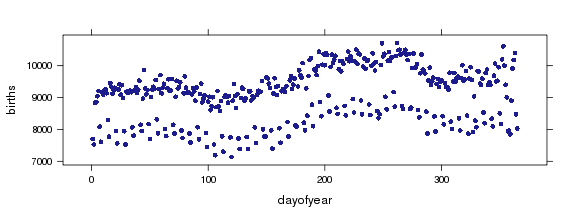### How do we make this plot?### How do we make this plot?### What is the Goal?

• a scatter plot

### What does R need to know?

• which variable goes where
• which data set

## xyplot (  births  ~  dayofyear  , data = Births78 )### Your turn: How do you make this plot?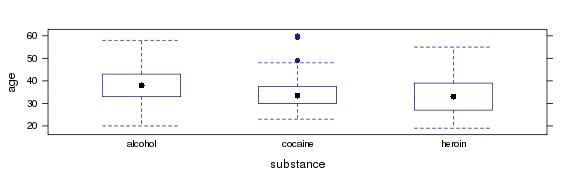### Your turn: How do you make this plot?The data: `HELPrct`

Variables: `age`, `substance`

Command: `bwplot()`

Raise your hand when you have created this plot

### Your turn: How do you make this plot?

``````bwplot( age ~ substance, data=HELPrct)
``````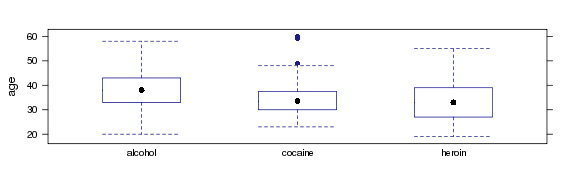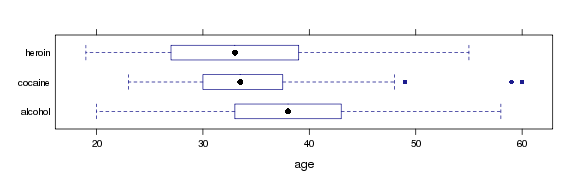Raise your hand when you have created this plot.

``````bwplot( substance ~ age, data=HELPrct )
``````### Graphical Summaries: One Variable

``````histogram( ~ age, data=HELPrct)
``````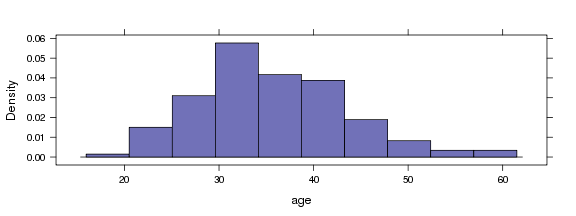Note: When there is one variable it is on the right side of the formula.

### One Variable

``````  histogram( ~age, data=HELPrct )
densityplot( ~age, data=HELPrct )
bwplot( ~age, data=HELPrct )
qqmath( ~age, data=HELPrct )
freqpolygon( ~age, data=HELPrct )
bargraph( ~sex, data=HELPrct )
``````

### Two Variables

``````xyplot(  i1 ~ age,       data=HELPrct )
bwplot( age ~ substance, data=HELPrct )
bwplot( substance ~ age, data=HELPrct )
``````
• i1 average number of drinks (standard units) consumed per day, in the past 30 days (measured at baseline)

### One variable

• `histogram()`, `qqmath()`, `densityplot()`, `freqpolygon()`, `bargraph()`

### Two Variables

• `xyplot()`, `bwplot()`

Create a plot of your own choosing with one of these data sets

``````names(KidsFeet)    # 4th graders' feet
?KidsFeet
``````
``````names(Utilities)   # utility bill data
?Utilities
``````
``````names(NHANES)      # body shape, etc.
?NHANES
``````

Type a question if you have trouble.

### groups and panels

• Add `groups =`group to overlay.
• Use `y ~ x | z` to create multipanel plots.
``````densityplot( ~ age | sex, data=HELPrct,
groups=substance,
auto.key=TRUE)
``````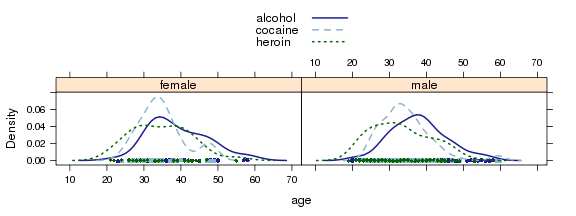### Bells & Whistles

• titles
• axis labels
• colors
• sizes
• transparency
• etc, etc.

My approach:

• Let the students ask or
• Let the data analysis drive

### Numerical Summaries: One Variable

Big idea:

• replace plot name with summary name
• nothing else changes
``````histogram( ~ age, data=HELPrct )
mean( ~ age, data=HELPrct )
``````
`````` 35.65
``````### Other Summaries

The mosaic package includes formula aware versions of `mean()`, `sd()`, `var()`, `min()`, `max()`, `sum()`, `IQR()`, …

Also provides `favstats()` to compute our favorites.

``````favstats( ~ age, data=HELPrct )
``````
`````` min Q1 median Q3 max  mean   sd   n missing
19 30     35 40  60 35.65 7.71 453       0
``````

### Tallying

``````tally( ~ sex, data=HELPrct)
``````
``````
female   male
107    346
``````
``````tally( ~ substance, data=HELPrct)
``````
``````
alcohol cocaine  heroin
177     152     124
``````

### Numerical Summaries: Two Variables

``````sd(   age ~ substance, data=HELPrct )
sd( ~ age | substance, data=HELPrct )
sd( ~ age, groups=substance, data=HELPrct )
``````
``````alcohol cocaine  heroin
7.652   6.693   7.986
``````

### Numerical Summaries: Tables

``````tally( sex ~ substance, data=HELPrct )
``````
``````        substance
sex      alcohol cocaine heroin
female  0.2034  0.2697 0.2419
male    0.7966  0.7303 0.7581
``````
``````tally( ~ sex + substance, data=HELPrct )
``````
``````        substance
sex      alcohol cocaine heroin
female      36      41     30
male       141     111     94
``````

### Numerical Summaries

``````mean( age ~ substance | sex, data=HELPrct,  )
``````
``````  A.F   C.F   H.F   A.M   C.M   H.M     F     M
39.17 34.85 34.67 37.95 34.36 33.05 36.25 35.47
``````
• I've abbreviated the names to make things fit on slide
• Also works for `median()`, `min()`, `max()`, `sd()`, `var()`, `favstats()`, etc.

### One Template to Rule a Lot

• single and multiple variable graphical summaries
• single and multiple variabble numerical summaries
• linear models
``````  mean( age ~ sex, data=HELPrct )
bwplot( age ~ sex, data=HELPrct )
lm( age ~ sex, data=HELPrct )
``````
``````female   male
36.25  35.47
``````
``````(Intercept)     sexmale
36.2523     -0.7841
``````

### Some other things

The `mosaic` package includes some other things, too

• Data sets (you've already seen some of them)
• xtras: `xchisq.test()`, `xpnorm()`, `xqqmath()`
• `mPlot()` – interactive plot design
• simplified `histogram()` controls (e.g., `width`)
• simplified ways to add onto lattice plots

### xpnorm()

``````xpnorm( 700, mean=500, sd=100)
``````
``````
If X ~ N(500,100), then

P(X <= 700) = P(Z <= 2) = 0.9772
P(X >  700) = P(Z >  2) = 0.0228
``````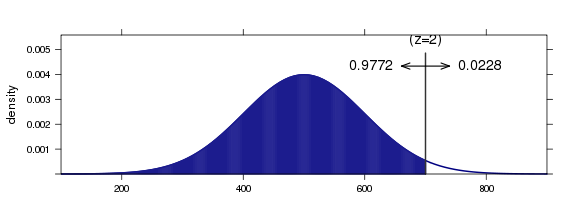`````` 0.9772
``````

### xpnorm()

``````xpnorm( c(300, 700), mean=500, sd=100)
``````
``````
If X ~ N(500,100), then

P(X <= 300) = P(Z <= -2) = 0.0228
P(X <= 700) = P(Z <= 2) = 0.9772
P(X >  300) = P(Z >  -2) = 0.9772
P(X >  700) = P(Z >  2) = 0.0228
``````